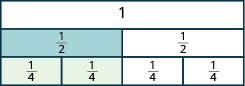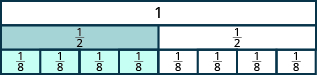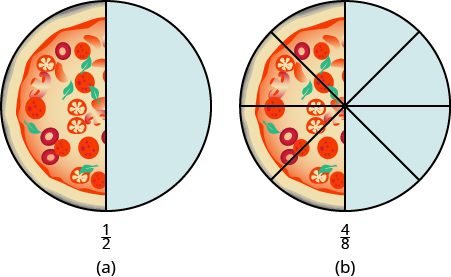## Modeling and Finding Equivalent Fractions

### Learning Outcomes

• Model equivalent fractions
• Find equivalent fractions

Let’s think about Andy and Bobby and their favorite food again. If Andy eats ${\Large\frac{1}{2}}$ of a pizza and Bobby eats ${\Large\frac{2}{4}}$ of the pizza, have they eaten the same amount of pizza? In other words, does ${\Large\frac{1}{2}}={\Large\frac{2}{4}}?$ We can use fraction tiles to find out whether Andy and Bobby have eaten equivalent parts of the pizza.

### Equivalent Fractions

Equivalent fractions are fractions that have the same value.

Fraction tiles serve as a useful model of equivalent fractions. You may want to use fraction tiles to do the following activity. Or you might make a copy of the fraction tiles shown earlier and extend it to include eighths, tenths, and twelfths.

Start with a ${\Large\frac{1}{2}}$ tile. How many fourths equal one-half? How many of the ${\Large\frac{1}{4}}$ tiles exactly cover the ${\Large\frac{1}{2}}$ tile?Since two ${\Large\frac{1}{4}}$ tiles cover the ${\Large\frac{1}{2}}$ tile, we see that ${\Large\frac{2}{4}}$ is the same as ${\Large\frac{1}{2}}$, or ${\Large\frac{2}{4}}={\Large\frac{1}{2}}$.

How many of the ${\Large\frac{1}{6}}$ tiles cover the ${\Large\frac{1}{2}}$ tile?Since three ${\Large\frac{1}{6}}$ tiles cover the ${\Large\frac{1}{2}}$ tile, we see that ${\Large\frac{3}{6}}$ is the same as ${\Large\frac{1}{2}}$.

So, ${\Large\frac{3}{6}}={\Large\frac{1}{2}}$. The fractions are equivalent fractions.

### Example

Use fraction tiles to find equivalent fractions. Show your result with a figure.

1. How many eighths (${\Large\frac{1}{8}}$) equal one-half (${\Large\frac{1}{2}}$)?
2. How many tenths (${\Large\frac{1}{10}}$) equal one-half (${\Large\frac{1}{2}}$)?
3. How many twelfths (${\Large\frac{1}{12}}$) equal one-half (${\Large\frac{1}{2}}$)?

Solution
1. It takes four ${\Large\frac{1}{8}}$ tiles to exactly cover the ${\Large\frac{1}{2}}$ tile, so ${\Large\frac{4}{8}}={\Large\frac{1}{2}}$.2. It takes five ${\Large\frac{1}{10}}$ tiles to exactly cover the ${\Large\frac{1}{2}}$ tile, so ${\Large\frac{5}{10}}={\Large\frac{1}{2}}$.3. It takes six ${\Large\frac{1}{12}}$ tiles to exactly cover the ${\Large\frac{1}{2}}$ tile, so ${\Large\frac{6}{12}}={\Large\frac{1}{2}}$.Suppose you had tiles marked ${\Large\frac{1}{20}}$. How many of them would it take to equal ${\Large\frac{1}{2}}$? Are you thinking ten tiles? If you are, you’re right, because ${\Large\frac{10}{20}}={\Large\frac{1}{2}}$.

We have shown that ${\Large\frac{1}{2},\frac{2}{4},\frac{3}{6},\frac{4}{8},\frac{5}{10},\frac{6}{12}}$, and ${\Large\frac{10}{20}}$ are all equivalent fractions.

## Find Equivalent Fractions

We used fraction tiles to show that there are many fractions equivalent to ${\Large\frac{1}{2}}$. For example, ${\Large\frac{2}{4},\frac{3}{6}}$, and ${\Large\frac{4}{8}}$ are all equivalent to ${\Large\frac{1}{2}}$. When we lined up the fraction tiles, it took four of the ${\Large\frac{1}{8}}$ tiles to make the same length as a ${\Large\frac{1}{2}}$ tile. This showed that ${\Large\frac{4}{8}}={\Large\frac{1}{2}}$. See the previous example.

We can show this with pizzas, too. Image (a) shows a single pizza, cut into two equal pieces with ${\Large\frac{1}{2}}$ shaded. Image (b) shows a second pizza of the same size, cut into eight pieces with ${\Large\frac{4}{8}}$ shaded.This is another way to show that ${\Large\frac{1}{2}}$ is equivalent to ${\Large\frac{4}{8}}$.

How can we use mathematics to change ${\Large\frac{1}{2}}$ into ${\Large\frac{4}{8}}$? How could you take a pizza that is cut into two pieces and cut it into eight pieces? You could cut each of the two larger pieces into four smaller pieces! The whole pizza would then be cut into eight pieces instead of just two. Mathematically, what we’ve described could be written as:

${\Large\frac{1\cdot\color{blue}{4}}{2\cdot\color{blue}{4}}}={\Large\frac{4}{8}}$

These models lead to the Equivalent Fractions Property, which states that if we multiply the numerator and denominator of a fraction by the same number, the value of the fraction does not change.

### Equivalent Fractions Property

If $a,b$, and $c$ are numbers where $b\ne 0$ and $c\ne 0$, then

${\Large\frac{a}{b}}={\Large\frac{a\cdot c}{b\cdot c}}$

When working with fractions, it is often necessary to express the same fraction in different forms. To find equivalent forms of a fraction, we can use the Equivalent Fractions Property. For example, consider the fraction one-half.

${\Large\frac{1\cdot\color{blue}{3}}{2\cdot\color{blue}{3}}}={\Large\frac{3}{6}}$ so ${\Large\frac{1}{2}}={\Large\frac{3}{6}}$

${\Large\frac{1\cdot\color{blue}{2}}{2\cdot\color{blue}{2}}}={\Large\frac{2}{4}}$ so ${\Large\frac{1}{2}}={\Large\frac{2}{4}}$

${\Large\frac{1\cdot\color{blue}{10}}{2\cdot\color{blue}{10}}}={\Large\frac{10}{20}}$ so ${\Large\frac{1}{2}}={\Large\frac{10}{20}}$

So, we say that ${\Large\frac{1}{2},\frac{2}{4},\frac{3}{6}}$, and ${\Large\frac{10}{20}}$ are equivalent fractions.

### Example

Find three fractions equivalent to ${\Large\frac{2}{5}}$.

### Try it

Find three fractions equivalent to ${\Large\frac{3}{5}}$.

Find three fractions equivalent to ${\Large\frac{4}{5}}$.

### Example

Find a fraction with a denominator of $21$ that is equivalent to ${\Large\frac{2}{7}}$.

### Try it

In the following video we show more examples of how to find an equivalent fraction given a specific denominator.

## Contribute!

Did you have an idea for improving this content? We’d love your input.